### Home > CAAC > Chapter 16 > Lesson 16.9.2.2 > Problem9-87

9-87.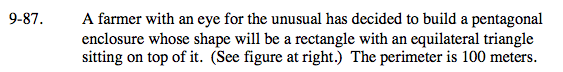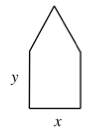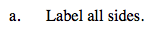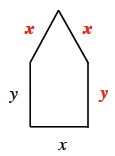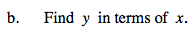2y + 3x = 100
2y = 100 − 3x

$y=\frac{100-3x}{2}$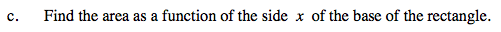Areatotal = Arearectangle + Areatriangle

To find the area of the triangle, drop an altitude forming two congruent triangles. The diagonal will be 'x'.

$\footnotesize \text{Use the Pythagorean Theorem to find the height. The area will be }\frac{(\text{height})(\text{base})}{2}.$